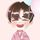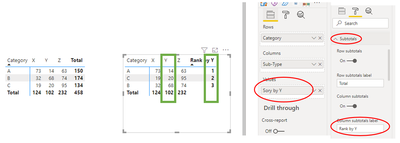cancel
Showing results for
Did you mean:Anonymous
Not applicable

## Pivot Chart sort by columns

is it possible to sort a pivot chart by column?

I just can higligh by clicking on them...

If not, any workaround?

1 ACCEPTED SOLUTIONCommunity Support

Hi @Anonymous ,

I have built a data sample. And according to my understanding, you may want to sort by X or Y or Z (Column Field) in matrix visual, right?Currently, it could easily sort by Category(Row Field)or Sub-Total in matrix as you seen, only these two fields has sort icon:In this case, you could replace the sub-total value by a measure to reach your requirement. Let's take how to sort by Y as an example:

1. Create a measure for Y sum:

``Y Sum = CALCULATE(SUM('Table'[Value]),FILTER(ALL('Table'),'Table'[Category]=MAX('Table'[Category]) && 'Table'[Sub-Type]="Y"))``

2. Use HASONEVALUE() , ISINCOPE() to change the sub-total value and use rank number to sort the Y sum :

``Sory by Y = IF(HASONEVALUE('Table'[Sub-Type]),SUM('Table'[Value]), IF(ISINSCOPE('Table'[Category]),RANKX(ALLSELECTED('Table'),[Y Sum],,ASC,Dense)))``

The final output is shown below:Best Regards,
Eyelyn Qin
If this post helps, then please consider Accept it as the solution to help the other members find it more quickly.

2 REPLIES 2Community Support

Hi @Anonymous ,

I have built a data sample. And according to my understanding, you may want to sort by X or Y or Z (Column Field) in matrix visual, right?Currently, it could easily sort by Category(Row Field)or Sub-Total in matrix as you seen, only these two fields has sort icon:In this case, you could replace the sub-total value by a measure to reach your requirement. Let's take how to sort by Y as an example:

1. Create a measure for Y sum:

``Y Sum = CALCULATE(SUM('Table'[Value]),FILTER(ALL('Table'),'Table'[Category]=MAX('Table'[Category]) && 'Table'[Sub-Type]="Y"))``

2. Use HASONEVALUE() , ISINCOPE() to change the sub-total value and use rank number to sort the Y sum :

``Sory by Y = IF(HASONEVALUE('Table'[Sub-Type]),SUM('Table'[Value]), IF(ISINSCOPE('Table'[Category]),RANKX(ALLSELECTED('Table'),[Y Sum],,ASC,Dense)))``

The final output is shown below:Best Regards,
Eyelyn Qin
If this post helps, then please consider Accept it as the solution to help the other members find it more quickly.Helper III

Hi @Anonymous ,

I think some screenshots and samples of the data and what are you trying to acheive would do great in understanding what are you after.

Regards

HAnnouncements#### Exclusive opportunity for Women!

Join us for a free, hands-on Microsoft workshop led by women trainers for women where you will learn how to build a Dashboard in a Day!#### Power Platform Conference-Power BI and Fabric Sessions

Join us Oct 1 - 6 in Las Vegas for the Microsoft Power Platform Conference.Top Solution Authors
Top Kudoed Authors
Users online (3,471)